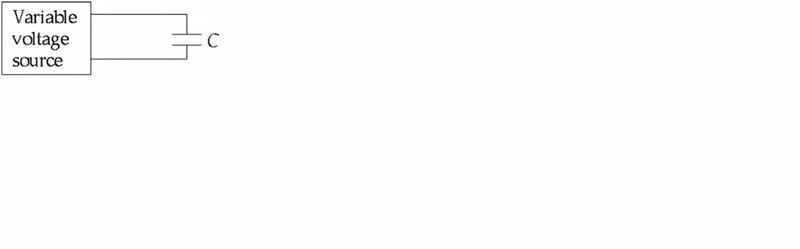# Variable Voltage Source

• Soaring Crane

## Homework Statement

As the voltage in the circuit shown below is increased (but not to the breakdown voltage), the capacitancea. increases.

b. decreases.

c. does not change.

d. increases, decreases, or does not change, depending on the charge on the plates of the capacitor.

e. does none of these.

## Homework Equations

C = Q/V or C = (epsilon_0*A)/(d)

## The Attempt at a Solution

If the voltage increases at a specific rate, then the charge would also increase since Q and V are directly proportional and the ratio between the two is a constant. Therefore, the capacitance does not change?

Thanks.

The capacitance should remain the same as it is a function of geometry and the material between the plates.

C = (epsilon * A) /d